# Writing a simple Compiler on my own - Implementing Register Allocation (part 3)[Custom Thumbnail]

All the Code of the series can be found at the Github repository:

https://github.com/drifter1/compiler

# Introduction

Hello it's a me again @drifter1! Today we continue with my Compiler Series, a series where we implement a complete compiler for a simple C-like language by using the C-tools Flex and Bison. In this article we will continue with the implementation of Register Allocation, getting into how we use the structures that we implemented in part 2.

## Requirements:

Actually you need to read and understand all the topics that I covered in the series as a whole, as these articles will give you access to knowledge about:
• What Compiler Design is (mainly the steps)
• For which exact Language the Compiler is build for (Tokens and Grammar)
• How to use Flex and Bison
• How to implement a lexer and parser for the language using those tools
• What the Symbol Table is and how we implement it
• How we combine Flex and Bison together
• How we can pass information from the Lexer to the Parser
• How we define operator priorities, precedencies and associativity
• What Semantic Analysis is (Attributes, SDT etc.)
• How we do the so called "Scope Resolution"
• How we declare types and check the type inter-compatibility for different cases that include function parameters and assignments
• How we check function calls later on using a "Revisit queue", as function declarations mostly happen after functions get used (in our Language)
• Intermediate Code Representations, including AST's
• How we implement an AST (structure and management)
• Action Rules for AST nodes and more
• The target system's architecture and its assembly language (MIPS)
• Register Allocation & Assignment and how we implement it
• How we generate Assembly code for various cases

## Difficulty:

Talking about the series in general this series can be rated:
Today's topic(s) can be rated:
• Medium

# Actual Tutorial Content

## Insert Variables into VarArray

Let's start this part with how we fill the first structure, which is the VarArray "var_name". This array has to contain the variables that we declare at the beginning of the main function and other temporary variables that occur through-out the code. Thus, this is the point where we have to decide the exact variables-values that have to be stored inside of registers, which is basically Register Allocation.

So, what has to be stored?

For the Simple C-like language that we are building we have to store the following:
• The variables that are being declared in the declarations-section
• Results of arithmetic, boolean, relational and equality operations
• Return values of function calls that are non-void
The first, can be found really easily looping through the declarations tree "main_decl_tree" and inserting the names of the decl-nodes. The rest are basically temporary variables that we insert at the point where we find an arithm, bool, rel, equ or non-void func_call node. We will name those variables "_temp", followed by the current "temp_count" value, increasing it afterwards. That way we will end up with _temp0, _temp1, etc.

As a diagram this can be summarized as: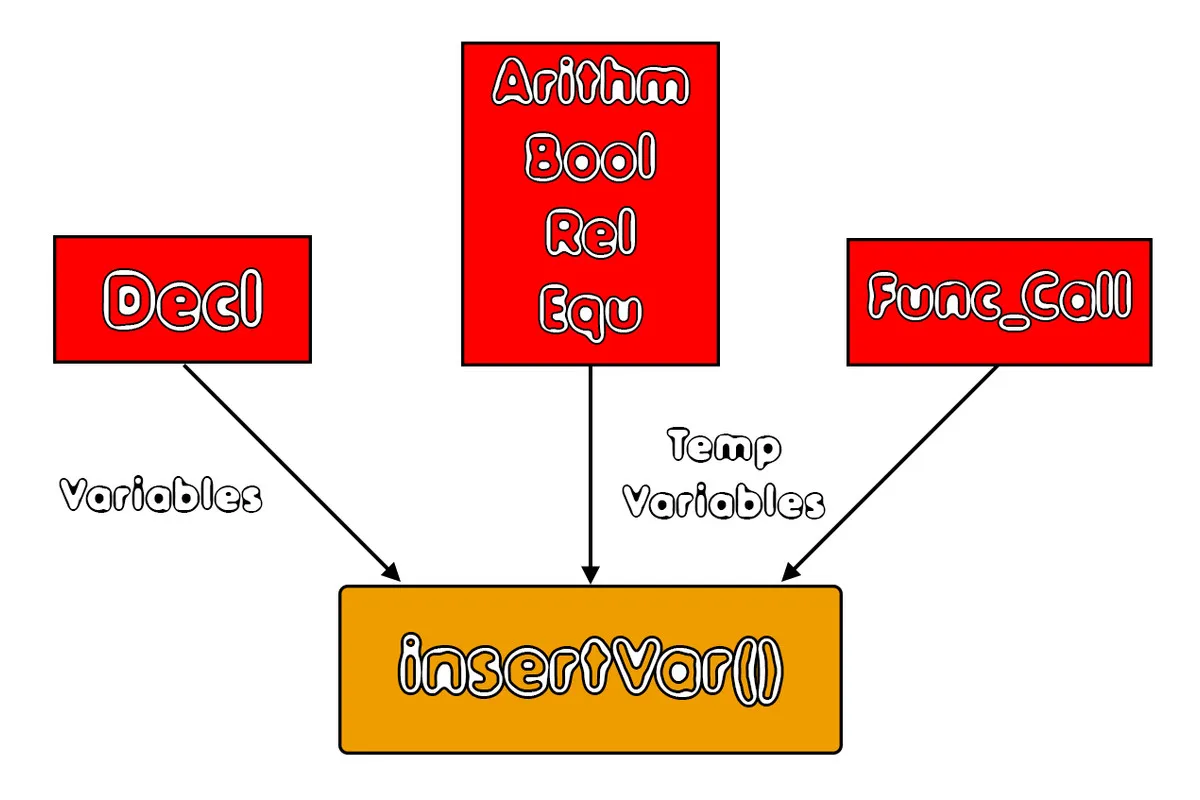To insert the declaration variables we need the following Code:
``````
AST_Node_Decl *temp_decl;
temp_decl = (struct AST_Node_Decl *) node;
for(i = 0; i < temp_decl->names_count; i++){
insertVar(temp_decl->names[i]->st_name);
}``````

To insert a temporary variable we need the following Code:
``````sprintf(name, "_temp%d", temp_count);
insertVar(name);
temp_count++;``````

These two things will have to be inside of the function "main_reg_allocation()", that will basically manage both the insertion into the VarArray and the insertion of Edges into the AdjGraph structure.

Now to the next structure, which helps us with Register Assignment. To be able to fill this structure, we have to decide which exact edges have to be inserted into the graph, meaning which exact values can't be stored in the same register inside of the same instruction (to keep things simpler - might optimize it more later though).

In our simple C-like Language, the things that can't be assigned to the same register are:
• The result and 2 (or 1) operand values in arithmetic, boolean, relation or equality instructions
• The assignment variable and assignment value in assignment statements
So, together with the creation of temporary variables inside of Arithm, Bool, Rel and Equ, we will also have to add 1 or 3 edges into the graph that tells us which variables are adjacent, and so can't have the same register. For assignments we will add one edge that tells us that the assignment entry can't have the same register as the assignment value, for which we can find the g_index using the custom function getGraphIndex(). This same function will also be used for the other cases.

Either way, enough explanation, let's get into each of them on its own...

### Arithmetic Nodes

Arithmetic operations are split into two categories:

#### 2 operands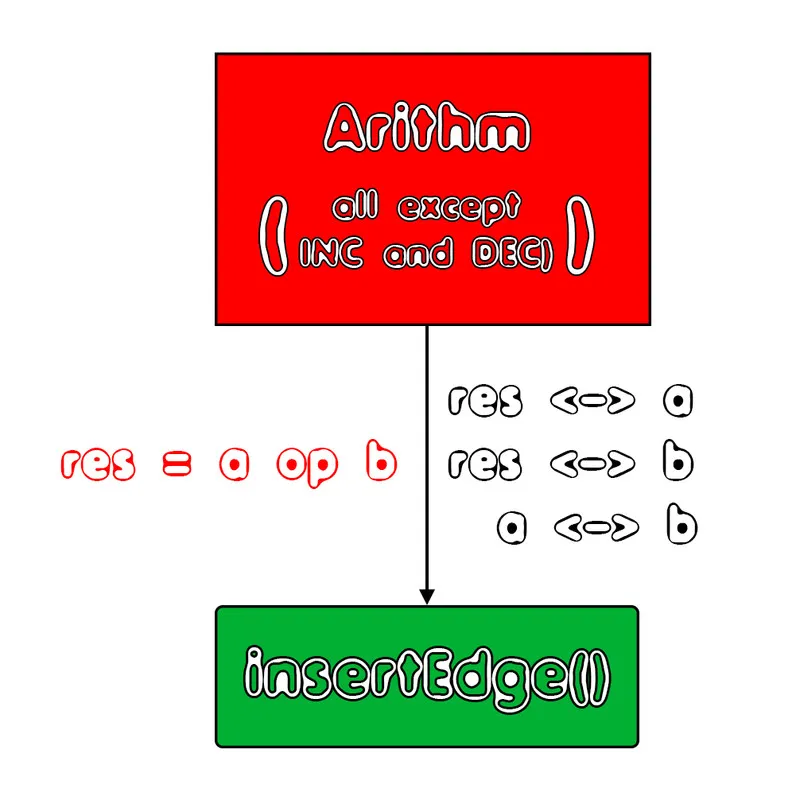#### 1 operand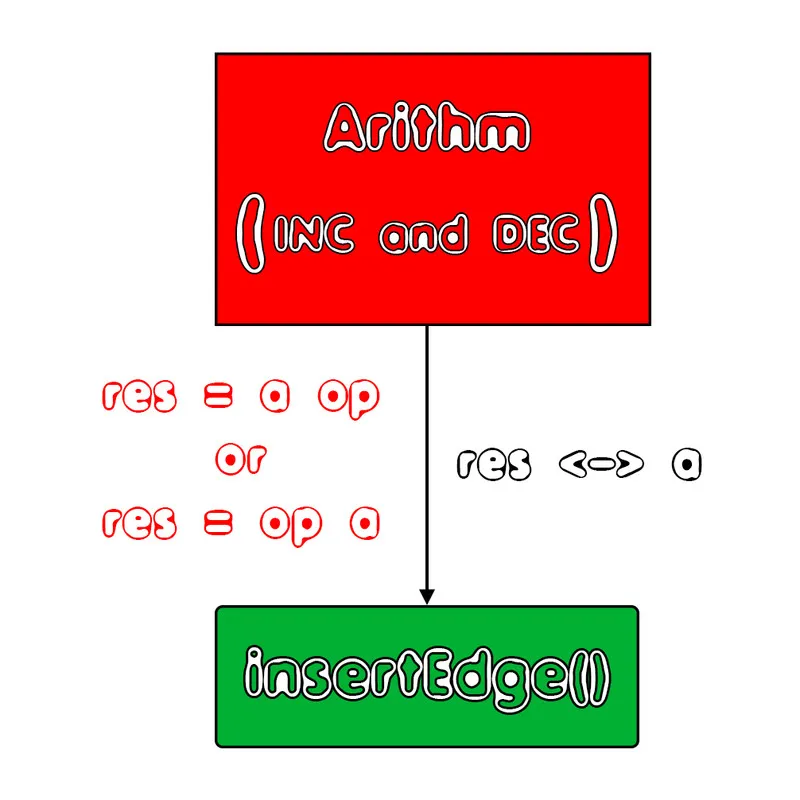As you can see we have to insert 3 edges in the case of 2 operands and 1 edge in the case of 1 single operand. If there is any constant inside of the operations, the g_index will be "-1" telling the insertEdge() function to not insert an Edge for that case.

In Code we will only have to write:
``````if(temp_arithm->op != INC && temp_arithm->op != DEC){
insertEdge(temp_arithm->g_index, getGraphIndex(temp_arithm->left));
insertEdge(temp_arithm->g_index, getGraphIndex(temp_arithm->right));
insertEdge(getGraphIndex(temp_arithm->left), getGraphIndex(temp_arithm->right));
}
else{
insertEdge(temp_arithm->g_index, getGraphIndex(temp_arithm->left));
}``````

### Boolean Nodes

Similaly, Boolean operations are also split into two categories:

#### 2 operands (AND or OR)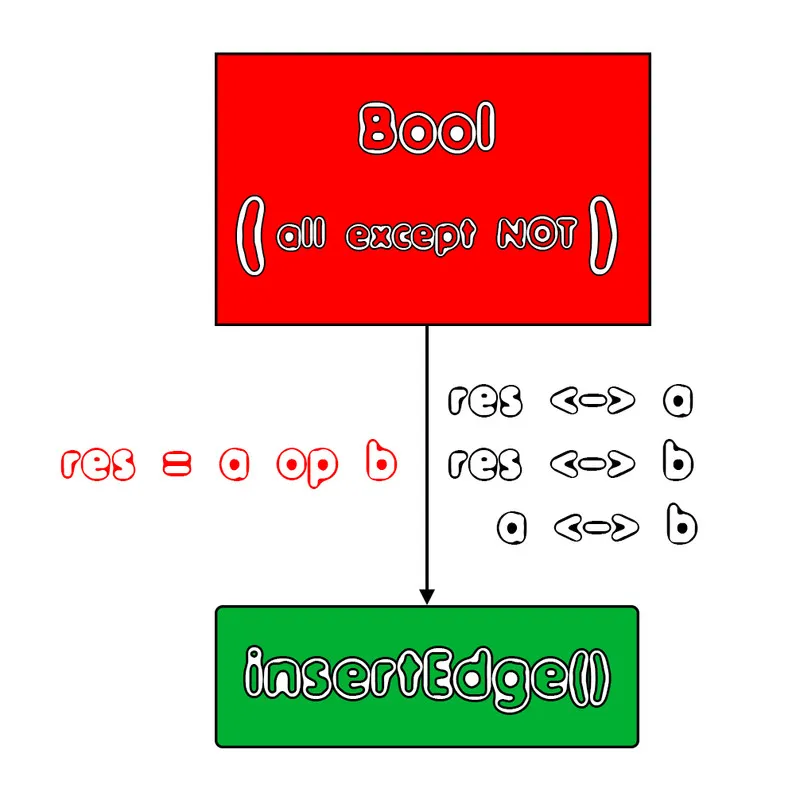#### 1 operand (NOT)In Code we only have to write:
``````if(temp_bool->op != NOT){
insertEdge(temp_bool->g_index, getGraphIndex(temp_bool->left));
insertEdge(temp_bool->g_index, getGraphIndex(temp_bool->right));
insertEdge(getGraphIndex(temp_bool->left), getGraphIndex(temp_bool->right));
}
else{
insertEdge(temp_bool->g_index, getGraphIndex(temp_bool->left));
}``````

### Relational-Equality Nodes

Relational and Equality Operators always have 2 operands: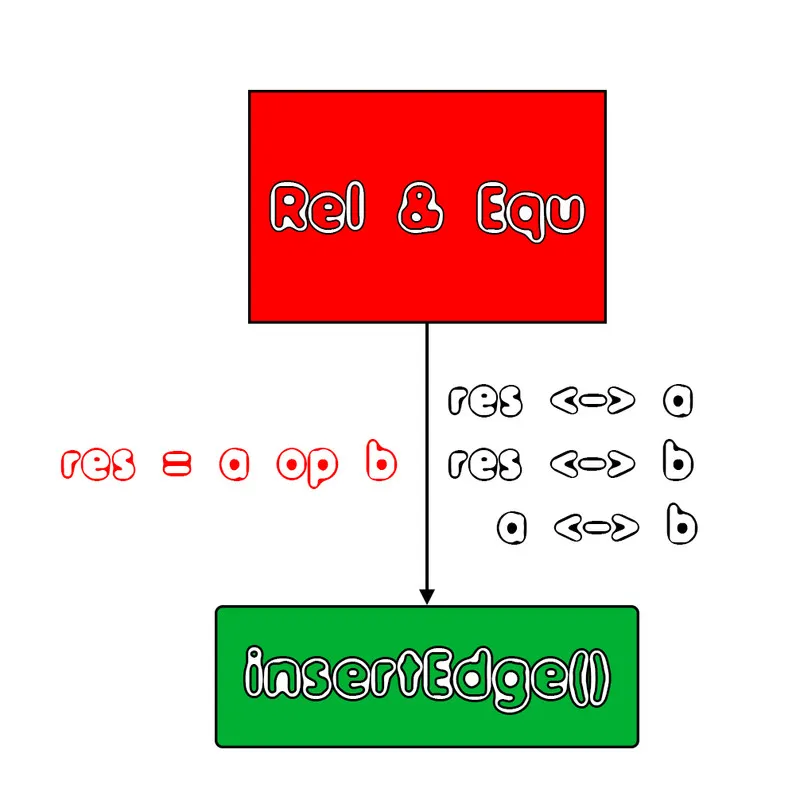For these cases we write the following Code:
``````Rel:
insertEdge(temp_rel->g_index, getGraphIndex(temp_rel->left));
insertEdge(temp_rel->g_index, getGraphIndex(temp_rel->right));
insertEdge(getGraphIndex(temp_rel->left), getGraphIndex(temp_rel->right));
----------------------------------------------------------------------------
Equ:
insertEdge(temp_equ->g_index, getGraphIndex(temp_equ->left));
insertEdge(temp_equ->g_index, getGraphIndex(temp_equ->right));
insertEdge(getGraphIndex(temp_equ->left), getGraphIndex(temp_equ->right));``````

### Assignment Nodes

In the case of assignments there is one adjacency to take care of: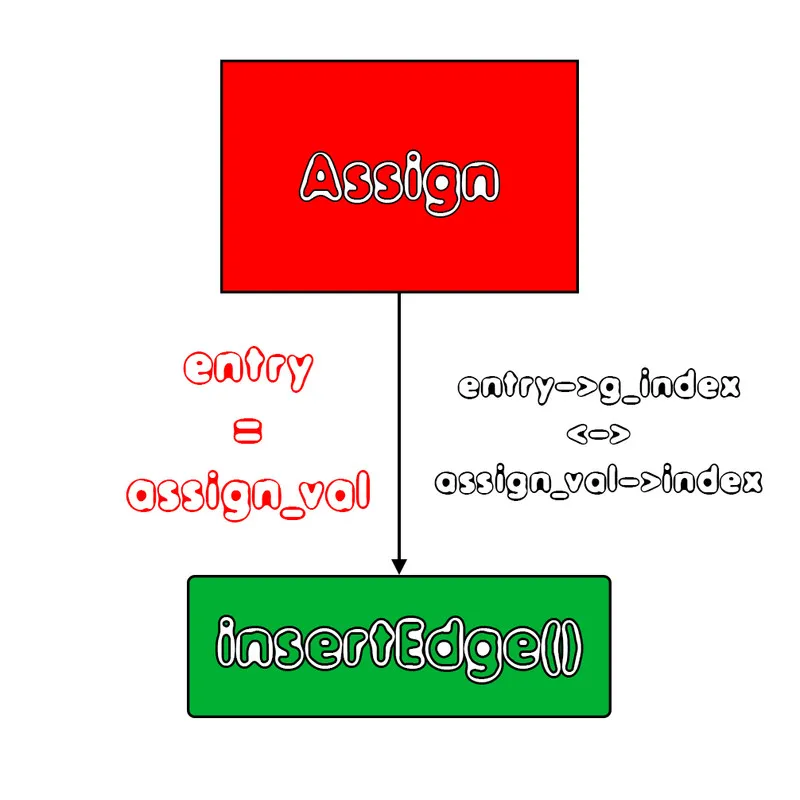The Code is only one simple line:
``insertEdge(temp_assign->entry->g_index, getGraphIndex(temp_assign->assign_val));``

## main_reg_allocation() Function

To fill the previously mentioned functions, we have to loop through each of the AST Trees. To do that we basically have to modify the ast_traversal() function that we used for printing, recursively looping through each "important" node of the trees and inserting variables & edges.

So, this function will be a switch-case that calls itself recursively, managing the various nodes depending on their node-type. We already explained how and when we insert variables or edges, so here is the code:
``````void main_reg_allocation(AST_Node *node){
static int inst_num = 0;
AST_Node_Declarations *temp_declarations;
AST_Node_Decl *temp_decl;
AST_Node_Arithm *temp_arithm;
AST_Node_Bool *temp_bool;
AST_Node_Rel *temp_rel;
AST_Node_Equ *temp_equ;
AST_Node_Statements *temp_statements;
AST_Node_If *temp_if;
AST_Node_Elsif *temp_elsif;
AST_Node_For *temp_for;
AST_Node_While *temp_while;
AST_Node_Incr *temp_incr;
AST_Node_Assign *temp_assign;
AST_Node_Func_Call *temp_func_call;
AST_Node_Call_Params *temp_call_params;
/* temp variable name */
char name[MAXTOKENLEN];
int i;
/* check if empty */
if(node == NULL){
return;
}
switch(node->type){
/* declarations case */
case DECLARATIONS:
temp_declarations = (struct AST_Node_Declarations *) node;
for(i = 0; i < temp_declarations->declaration_count; i++){
main_reg_allocation(temp_declarations->declarations[i]);
}
break;
/* declaration case */
case DECL_NODE:
temp_decl = (struct AST_Node_Decl *) node;
for(i = 0; i < temp_decl->names_count; i++){
insertVar(temp_decl->names[i]->st_name);
/* graph index */
temp_decl->names[i]->g_index = getVarIndex(temp_decl->names[i]->st_name);
}
break;
/* left and right child cases */
case BASIC_NODE:
main_reg_allocation(node->left);
main_reg_allocation(node->right);
break;
case ARITHM_NODE:
temp_arithm = (struct AST_Node_Arithm *) node;
main_reg_allocation(node->left);
main_reg_allocation(node->right);
/* insert temporary */
sprintf(name, "_temp%d", temp_count);
insertVar(name);
temp_count++;
declare = 1;
insert(name, strlen(name), temp_arithm->data_type, -1);
declare = 0;
/* graph index */
temp_arithm->g_index = var_count - 1;
/* manage graph */
if(temp_arithm->op != INC && temp_arithm->op != DEC){
insertEdge(temp_arithm->g_index, getGraphIndex(temp_arithm->left));
insertEdge(temp_arithm->g_index, getGraphIndex(temp_arithm->right));
insertEdge(getGraphIndex(temp_arithm->left), getGraphIndex(temp_arithm->right));
}
else{
insertEdge(temp_arithm->g_index, getGraphIndex(temp_arithm->left));
}
inst_num++;
break;
case BOOL_NODE:
temp_bool = (struct AST_Node_Bool *) node;
main_reg_allocation(node->left);
main_reg_allocation(node->right);
/* insert temporary */
sprintf(name, "_temp%d", temp_count);
insertVar(name);
temp_count++;
declare = 1;
insert(name, strlen(name), temp_bool->data_type, -1);
declare = 0;
/* graph index */
temp_bool->g_index = var_count - 1;
/* manage graph */
if(temp_bool->op != NOT){
insertEdge(temp_bool->g_index, getGraphIndex(temp_bool->left));
insertEdge(temp_bool->g_index, getGraphIndex(temp_bool->right));
insertEdge(getGraphIndex(temp_bool->left), getGraphIndex(temp_bool->right));
}
else{
insertEdge(temp_bool->g_index, getGraphIndex(temp_bool->left));
}
inst_num++;
break;
case REL_NODE:
temp_rel = (struct AST_Node_Rel *) node;
main_reg_allocation(node->left);
main_reg_allocation(node->right);
/* insert temporary */
sprintf(name, "_temp%d", temp_count);
insertVar(name);
temp_count++;
declare = 1;
insert(name, strlen(name), temp_rel->data_type, -1);
declare = 0;
/* graph index */
temp_rel->g_index = var_count - 1;
/* manage graph */
insertEdge(temp_rel->g_index, getGraphIndex(temp_rel->left));
insertEdge(temp_rel->g_index, getGraphIndex(temp_rel->right));
insertEdge(getGraphIndex(temp_rel->left), getGraphIndex(temp_rel->right));
inst_num++;
break;
case EQU_NODE:
temp_equ = (struct AST_Node_Equ *) node;
main_reg_allocation(node->left);
main_reg_allocation(node->right);
/* insert temporary */
sprintf(name, "_temp%d", temp_count);
insertVar(name);
temp_count++;
declare = 1;
insert(name, strlen(name), temp_equ->data_type, -1);
declare = 0;
/* graph index */
temp_equ->g_index = var_count - 1;
/* manage graph */
insertEdge(temp_equ->g_index, getGraphIndex(temp_equ->left));
insertEdge(temp_equ->g_index, getGraphIndex(temp_equ->right));
insertEdge(getGraphIndex(temp_equ->left), getGraphIndex(temp_equ->right));
inst_num++;
break;
/* reference case */
case REF_NODE:
/* all the entries are already being managed by the Decl case */
break;
/* constant case */
case CONST_NODE:
/* already managed in getGraphIndex */
break;
/* statements case */
case STATEMENTS:
temp_statements = (struct AST_Node_Statements *) node;
for(i = 0; i < temp_statements->statement_count; i++){
main_reg_allocation(temp_statements->statements[i]);
}
break;
/* the if case */
case IF_NODE:
temp_if = (struct AST_Node_If *) node;
main_reg_allocation(temp_if->condition);
inst_num++;
main_reg_allocation(temp_if->if_branch);
if(temp_if->elseif_count > 0 ){
for(i = 0; i < temp_if->elseif_count; i++){
main_reg_allocation(temp_if->elsif_branches[i]);
}
}
if(temp_if->else_branch != NULL){
main_reg_allocation(temp_if->else_branch);
}
break;
/* the else if case */
case ELSIF_NODE:
temp_elsif = (struct AST_Node_Elsif *) node;
main_reg_allocation(temp_elsif->condition);
inst_num++;
main_reg_allocation(temp_elsif->elsif_branch);
break;
/* for case */
case FOR_NODE:
temp_for = (struct AST_Node_For *) node;
main_reg_allocation(temp_for->initialize);
inst_num++;
main_reg_allocation(temp_for->condition);
inst_num++;
main_reg_allocation(temp_for->for_branch);
main_reg_allocation(temp_for->increment);
inst_num++;
break;
/* while case */
case WHILE_NODE:
temp_while = (struct AST_Node_While *) node;
main_reg_allocation(temp_while->condition);
inst_num++;
main_reg_allocation(temp_while->while_branch);
break;
/* assign case */
case ASSIGN_NODE:
temp_assign = (struct AST_Node_Assign *) node;
/* manage graph */
insertEdge(temp_assign->entry->g_index, getGraphIndex(temp_assign->assign_val));
main_reg_allocation(temp_assign->assign_val);
break;
/* simple case */
case SIMPLE_NODE:
inst_num++;
break;
/* increment statement */
case INCR_NODE:
temp_incr = (AST_Node_Incr*) node;
inst_num++;
break;
/* function call case */
case FUNC_CALL:
temp_func_call = (struct AST_Node_Func_Call *) node;
if(temp_func_call->num_of_pars != 0){
for(i = 0; i < temp_func_call->num_of_pars; i++){
main_reg_allocation(temp_func_call->params[i]);
}
}
/* insert temporary when function non-void */
if(temp_func_call->entry->inf_type != VOID_TYPE){
sprintf(name, "_temp%d", temp_count);
insertVar(name);
temp_count++;
declare = 1;
insert(name, strlen(name), temp_func_call->entry->inf_type, -1);
declare = 0;
/* graph index */
temp_func_call->g_index = var_count - 1;
}
inst_num++;
break;
case CALL_PARAMS:
temp_call_params = (struct AST_Node_Call_Params*) node;
if(temp_call_params->num_of_pars > 0){
for(i = 0; i < temp_call_params->num_of_pars; i++){
main_reg_allocation(temp_call_params->params[i]);
}
}
break;
/* function declaration stuff */
case FUNC_DECLS:
case FUNC_DECL:
case RET_TYPE:
case DECL_PARAMS:
case RETURN_NODE:
/* can't occur in main */
break;
default: /* wrong choice case */
fprintf(stderr, "Error in node selection!\n");
exit(1);
}
}``````

Where this funcion gets called and how we do the actual Register Assignment using Graph Coloring will be covered in the next part...

## RESOURCES

### References:

No references, just using code that I implemented in my previous articles.

### Images:

All of the images are custom-made!

## Final words | Next up on the project

And this is actually it for today's post! I hope that I explained everything as much as I needed to. Next time we will continue with part 4.

Next up on this series, in general, are:
• Machine Code generation (MIPS Assembly)
• Various Optimizations in the Compiler's Code
Which are all topics for which we will need more than one article to complete them. Also, note that we might also get into AST Code Optimizations later on, or that we could even extend the Language by adding complex datatypes (structs and unions), more rules etc.

So, see ya next time!

## GitHub Account:

https://github.com/drifter1Keep on drifting! ;)

H2
H3
H4
3 columns
2 columns
1 column